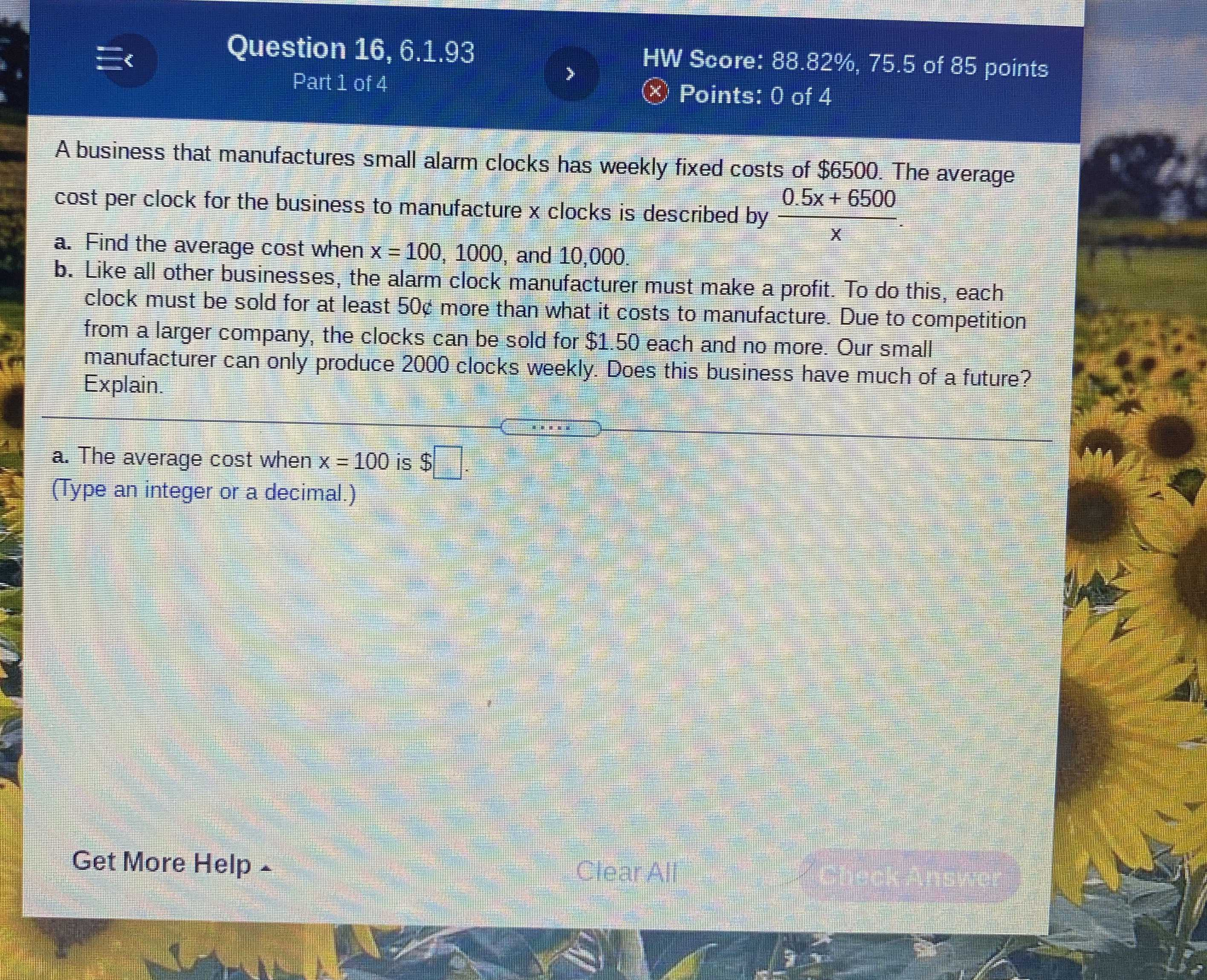### Still have math questions?

Algebra
QuestionA business that manufactures small alarm clocks has weekly fixed costs of $$\ 6500$$ . The average cost per clock for the business to manufacture $$x$$ clocks is described by $$\frac { 0.5 x + 6500 } { x }$$.

a. Find the average cost when $$x = 100,1000$$ , and $$10,000$$ .

b. Like all other businesses, the alarm clock manufacturer must make a profit. To do this, each clock must be sold for at least $$50 \%$$ more than what it costs to manufacture. Due to competition from a larger company, the clocks can be sold for $$\ 1.50$$ each and no more. Our small manufacturer can only produce $$2000$$ clocks weekly. Does this business have much of a future? Explain.

a. The average cost when $$x = 100$$ is $$\ \square$$ .

(Type an integer or a decimal.)